# Statistical analysis of sensor data#

These tutorials describe some approaches to statistical analysis of sensor-level data.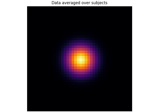Statistical inference

Statistical inference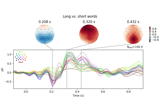Visualising statistical significance thresholds on EEG data

Visualising statistical significance thresholds on EEG data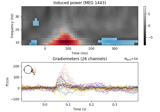Non-parametric 1 sample cluster statistic on single trial power

Non-parametric 1 sample cluster statistic on single trial power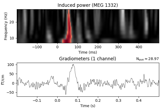Non-parametric between conditions cluster statistic on single trial power

Non-parametric between conditions cluster statistic on single trial power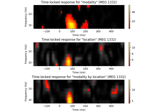Mass-univariate twoway repeated measures ANOVA on single trial power

Mass-univariate twoway repeated measures ANOVA on single trial power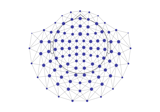Spatiotemporal permutation F-test on full sensor data

Spatiotemporal permutation F-test on full sensor data